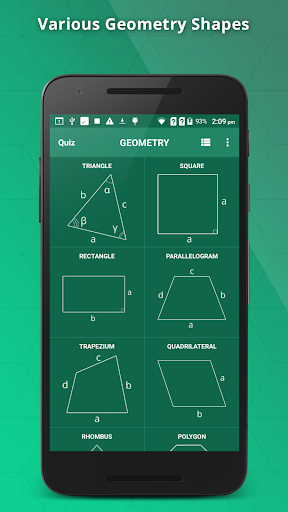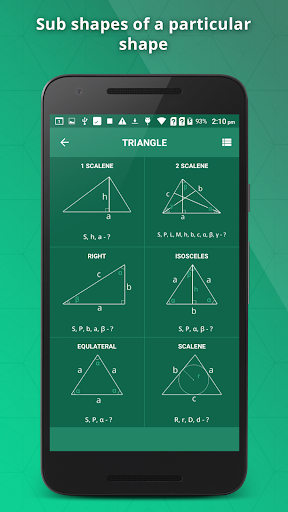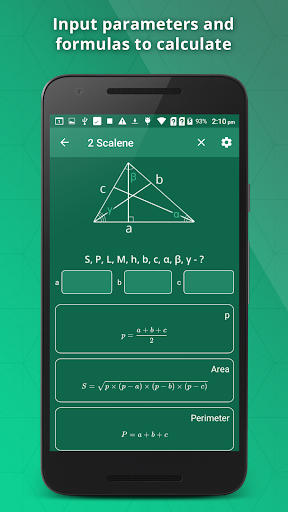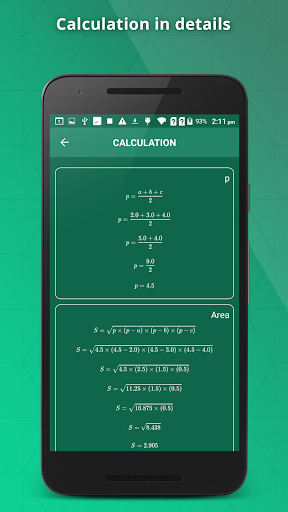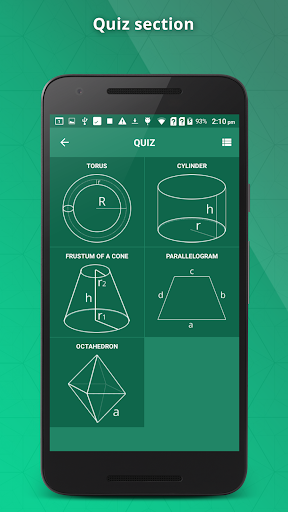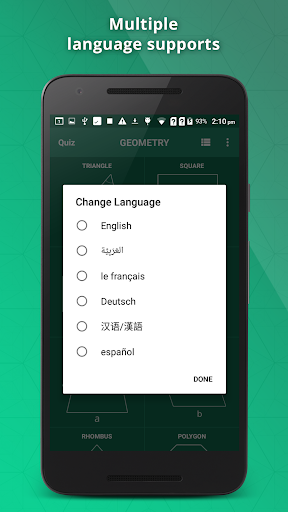GeometrySoln is Geometry Calculator for Students and Teachers, which makes Geometry Calculations very Easy and Quick. From Basic Calculation of Perimeter of a Square, to Calculating a Volume of a Cube, you can get it all. It displays the formulas to calculate the same and also it will calculate, the Perimeter, Area, Volume, etc, It will also display a Detailed Calculation Steps on how the result was obtained.

You can work with Following Shapes

Square
Triangle
Rectangle
Parallelogram
Trapezium
Rhombus
Polygon
Circle
Annulus
Ellipse
Torus
Ball
Cube
Parallelepiped
Cylinder
Cone
Prism
Pyramid
Octahedron
Capsule
Hemisphere
Rectangular Prism
Tube